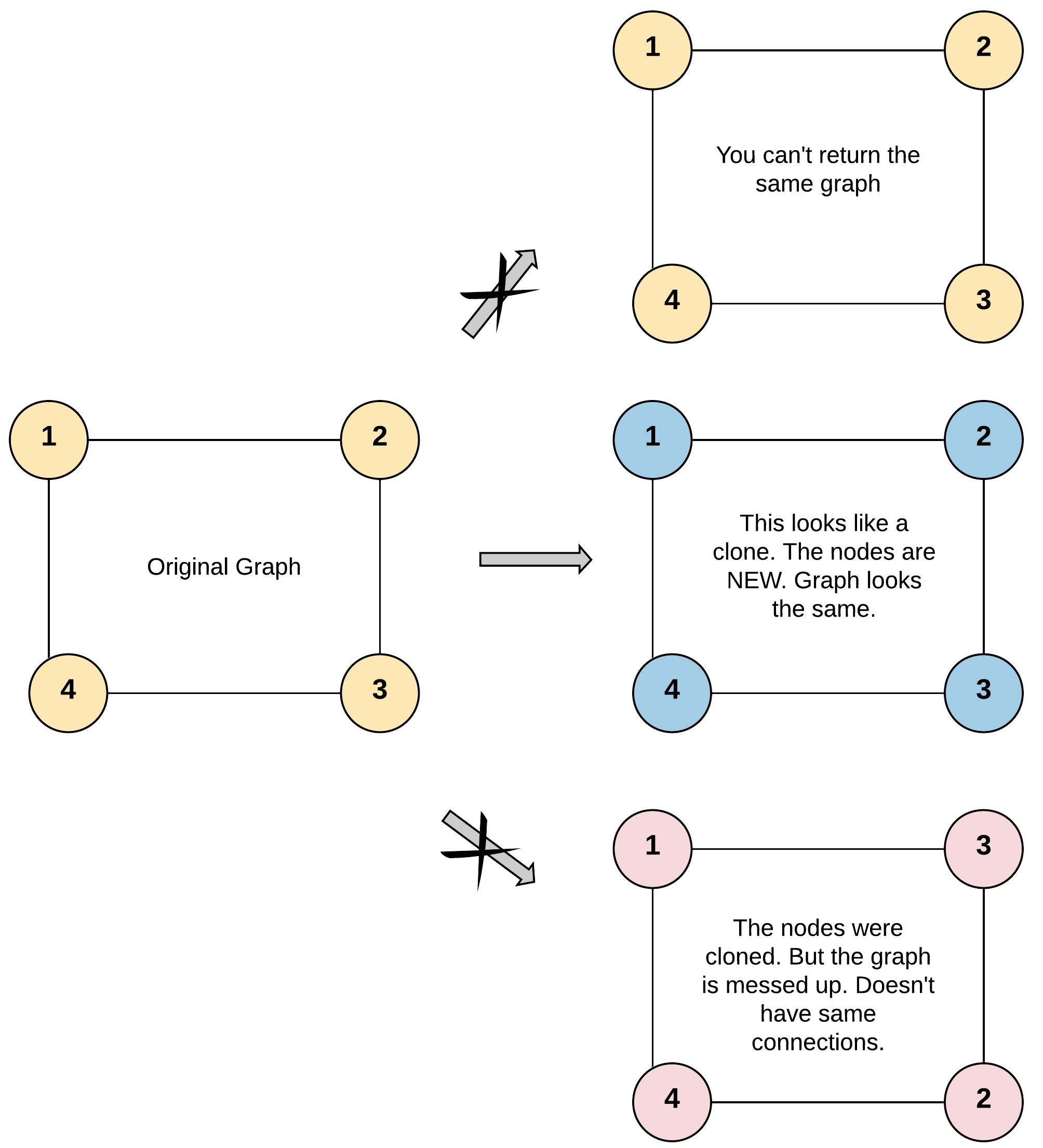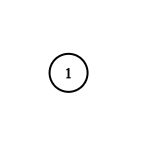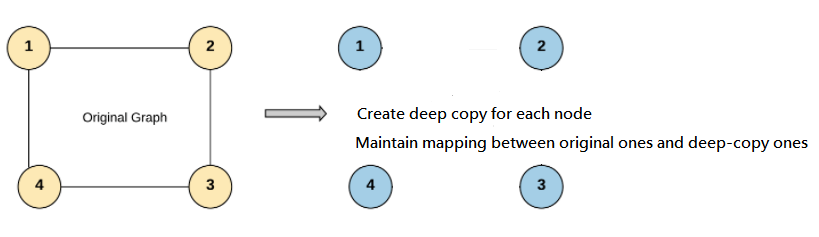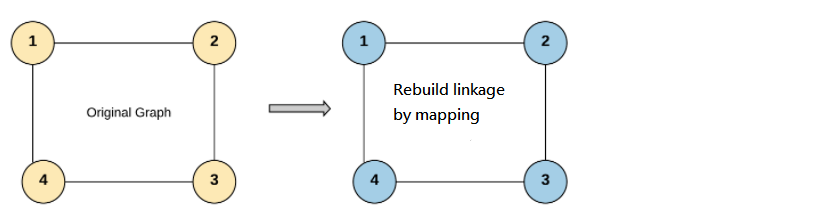Given a reference of a node in a connected undirected graph.

Return a deep copy (clone) of the graph.

Each node in the graph contains a val (int) and a list (List[Node]) of its neighbors.

class Node {
public int val;
public List<Node> neighbors;
}

Test case format:

For simplicity sake, each node’s value is the same as the node’s index (1-indexed). For example, the first node with val = 1, the second node with val = 2, and so on. The graph is represented in the test case using an adjacency list.

Adjacency list is a collection of unordered lists used to represent a finite graph. Each list describes the set of neighbors of a node in the graph.

The given node will always be the first node with val = 1. You must return the copy of the given node as a reference to the cloned graph.

Example 1:Output: [[2,4],[1,3],[2,4],[1,3]]
Explanation: There are 4 nodes in the graph.
1st node (val = 1)'s neighbors are 2nd node (val = 2) and 4th node (val = 4).
2nd node (val = 2)'s neighbors are 1st node (val = 1) and 3rd node (val = 3).
3rd node (val = 3)'s neighbors are 2nd node (val = 2) and 4th node (val = 4).
4th node (val = 4)'s neighbors are 1st node (val = 1) and 3rd node (val = 3).

Example 2:Output: [[]]
Explanation: Note that the input contains one empty list. The graph consists of only one node with val = 1 and it does not have any neighbors.

Example 3:

Output: []
Explanation: This an empty graph, it does not have any nodes.

Example 4:Output: [,]

Constraints:

• 1 <= Node.val <= 100
• Node.val is unique for each node.
• Number of Nodes will not exceed 100.
• There is no repeated edges and no self-loops in the graph.
• The Graph is connected and all nodes can be visited starting from the given node.

Recursive DFS Solution [w/ Diagram]

Review:

This one is very similar to Leetcode #138 Copy List with Random Pointer

The key idea behind is the same:

1. Create deep copy for each node, and maintain the mapping relation2. Rebuild node linkage by mapping relationHint:

Stage_1:

Create deep copy for each node in DFS
Maintain a mapping relation (i.e., hash map or dictionary) between original ones and deep-copy ones.

Stage_2:

Rebuild neighbor list in DFS with mapping relation.

Implementation:

class Solution:
def cloneGraph(self, node: 'Node') -> 'Node':

# key: memory id of original node
# value: corresponding deep copy node
mapping = {}

# -----------------------------------------
def helper( node: 'Node' ) -> 'Node':

if not node:

# empty node's deep copy is still empty node
return node

elif id(node) in mapping:

# current node already has deep copy
return mapping[ id(node) ]

# create deep copy for current node
mapping[ id(node) ] = Node( val=node.val, neighbors=[] )

for original_neighbor in node.neighbors:
# update neighbor list for current node
mapping[ id(node) ].neighbors.append( helper(original_neighbor) )

return  mapping[ id(node) ]

# -----------------------------------------
return helper( node )

RECURSIVE DFS

"""
# Definition for a Node.
class Node:
def __init__(self, val = 0, neighbors = None):
self.val = val
self.neighbors = neighbors if neighbors is not None else []
"""

class Solution:
def cloneGraph(self, node: 'Node') -> 'Node':
def dfs(curr):
if not curr: return curr

if curr in visited:
return visited[curr]

clone = Node(curr.val,[])

visited[curr] = clone

if curr.neighbors:
clone.neighbors = [dfs(n) for n in curr.neighbors]

return clone

visited = {}
return dfs(node)

Python BFS

"""
# Definition for a Node.
class Node:
def __init__(self, val = 0, neighbors = None):
self.val = val
self.neighbors = neighbors if neighbors is not None else []
"""

class Solution:
def cloneGraph(self, node: 'Node') -> 'Node':
if not node:
return node

visited = {}
queue = deque([node])
visited[node] = Node(node.val, [])

while queue:
n = queue.popleft()

for neighbor in n.neighbors:
if neighbor not in visited:
visited[neighbor] = Node(neighbor.val, [])
queue.append(neighbor)
visited[n].neighbors.append(visited[neighbor])

return visited[node]
Previous Post

Next article